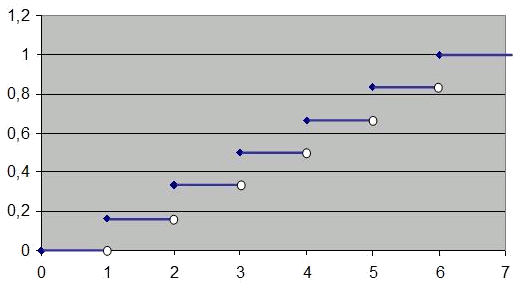# The distribution function

The distribution function of a random variable $$X$$ is a function that assigns, for every point, the probability accumulated up to the above mentioned value. That is:

$$F(X)=p(X \leq x)$$\$

For example, we will compute the distribution function of the random variable $$X$$, resulting from throwing a perfect dice.

The following table shows the values of $$F (x)$$:

 x F (x) $$x < 1$$ $$0$$ $$1\leq x < 2$$ $$\displaystyle \frac{1}{6}$$ $$1\leq x < 3$$ $$\displaystyle \frac{2}{6}$$ $$1\leq x < 4$$ $$\displaystyle \frac{3}{6}$$ $$1\leq x < 5$$ $$\displaystyle \frac{4}{6}$$ $$1\leq x < 6$$ $$\displaystyle \frac{5}{6}$$ $$x \leq 6$$ $$1$$

The value of the function distribution in $$-\infty$$ will always be $$0$$, while the value in $$+\infty$$ will always be $$1$$.

This turns out to be quite intuitive, since the probability that the value of $$x$$ is smaller than $$-\infty$$ is zero, and the probability that it is less than $$+\infty$$ is $$1$$ (since it is always less or equal to $$+\infty$$).Since it is a discrete random variable, the distribution function will be discrete. Note that the probability of obtaining a result lower than $$5,2$$ is the same as that of lower than $$5,3$$ or $$5,9$$.